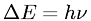Equations > Chemistry > Atomic Structure > Planck's Quantized (Quantum) Energy Equation

### Planck's Quantized (Quantum) Energy EquationLatex Code:

MathML Code:

 $\Delta E=h\nu$

MathType 5.0: